# RunKit +#### Try any Node.js package right in your browser

This is a `playground` to test code. It runs a full `Node.js` environment and already has all of `npm`’s 400,000 packages pre-installed, including `ngraph.generators` with all `npm` packages installed. Try it out:

var ngraphGenerators = require("ngraph.generators")

This service is provided by RunKit and is not affiliated with npm, Inc or the package authors.

# Graph generators

This module generates various graphs. It is part of larger ngraph family. If you need something not as simple as generated graphs, please check out ngraph.sparce-collection repository which contains graphs from University of Florida collection.# Supported graphs

All images below are clickable and point to interactive 3d visualization, done by `ngraph.three` library.

``````// Creates a ladder with 10 steps
var graph = require('ngraph.generators').ladder(10);
``````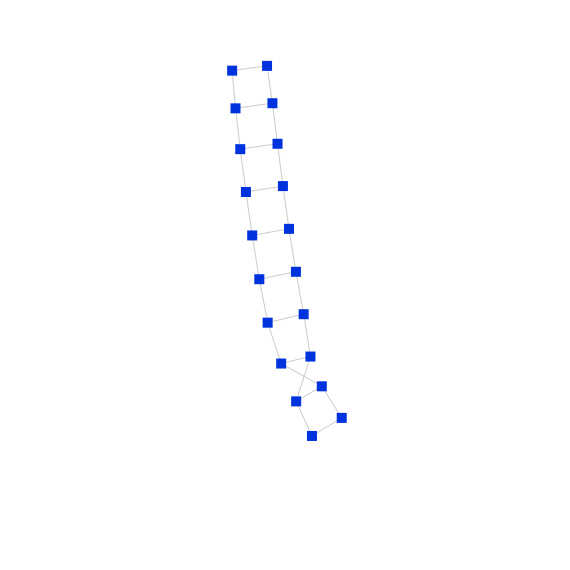## complete

``````// Creates complete graph K6
var graph = require('ngraph.generators').complete(6);
``````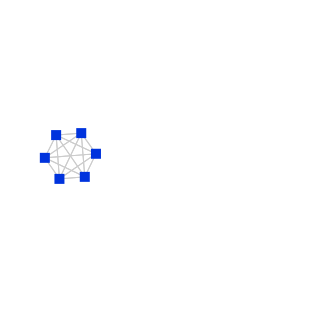## completeBipartite

``````// Creates complete bipartite graph K 3,3.
var graph = require('ngraph.generators').completeBipartite(3, 3);
``````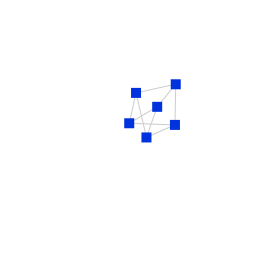## balancedBinTree

``````// Creates balanced binary tree with n levels.
var graph = require('ngraph.generators').balancedBinTree(5);
``````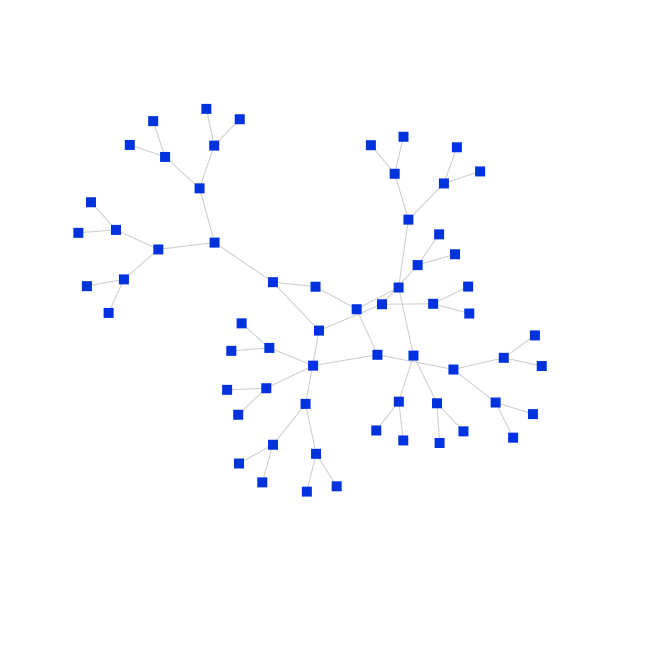## path

``````// Generates a path-graph with 10 steps.
var graph = require('ngraph.generators').path(10);
``````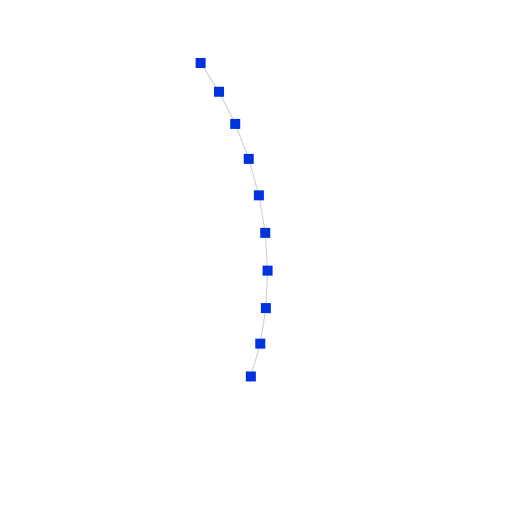``````// Generates a graph in a form of a circular ladder with 5 steps.
var graph = require('ngraph.generators').circularLadder(5);
``````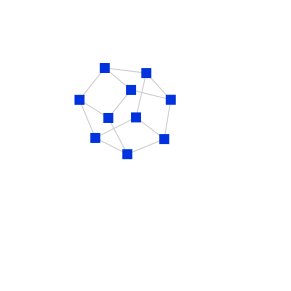## grid

``````// Generates a graph in a form of a grid with 10 rows and 10 columns.
var graph = require('ngraph.generators').grid(10, 10);
``````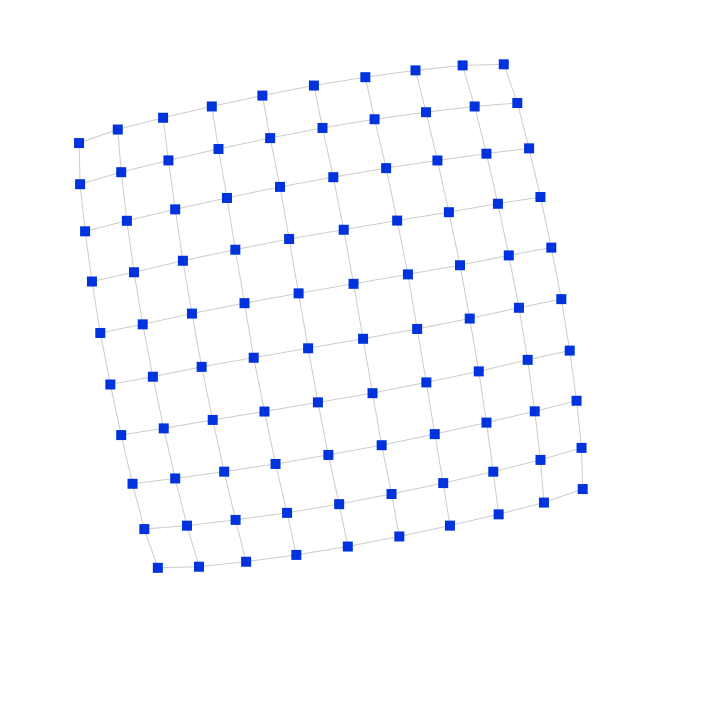## grid3

``````// Generates a graph in a form of a 3d grid with 5 rows and 5 columns and 5 levels.
var graph = require('ngraph.generators').grid3(5, 5, 5);
``````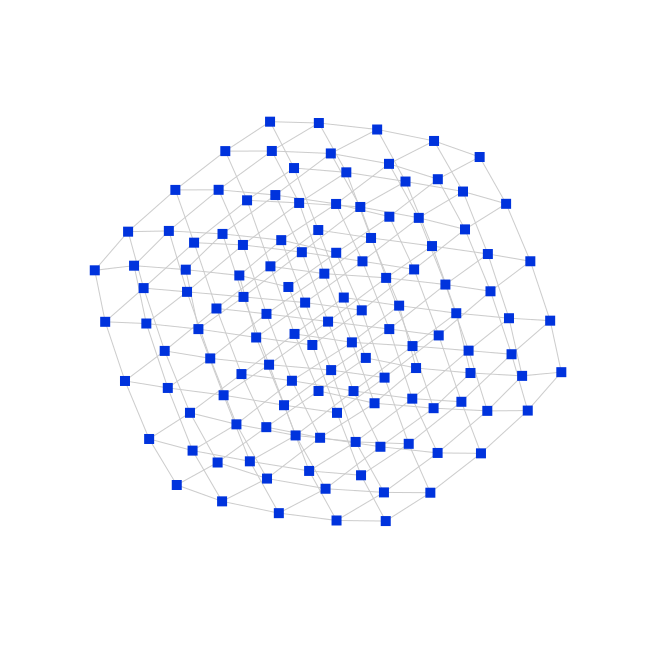``````// Creates graph with 100 nodes and 0 links
var graph = require('ngraph.generators').noLinks(100);
``````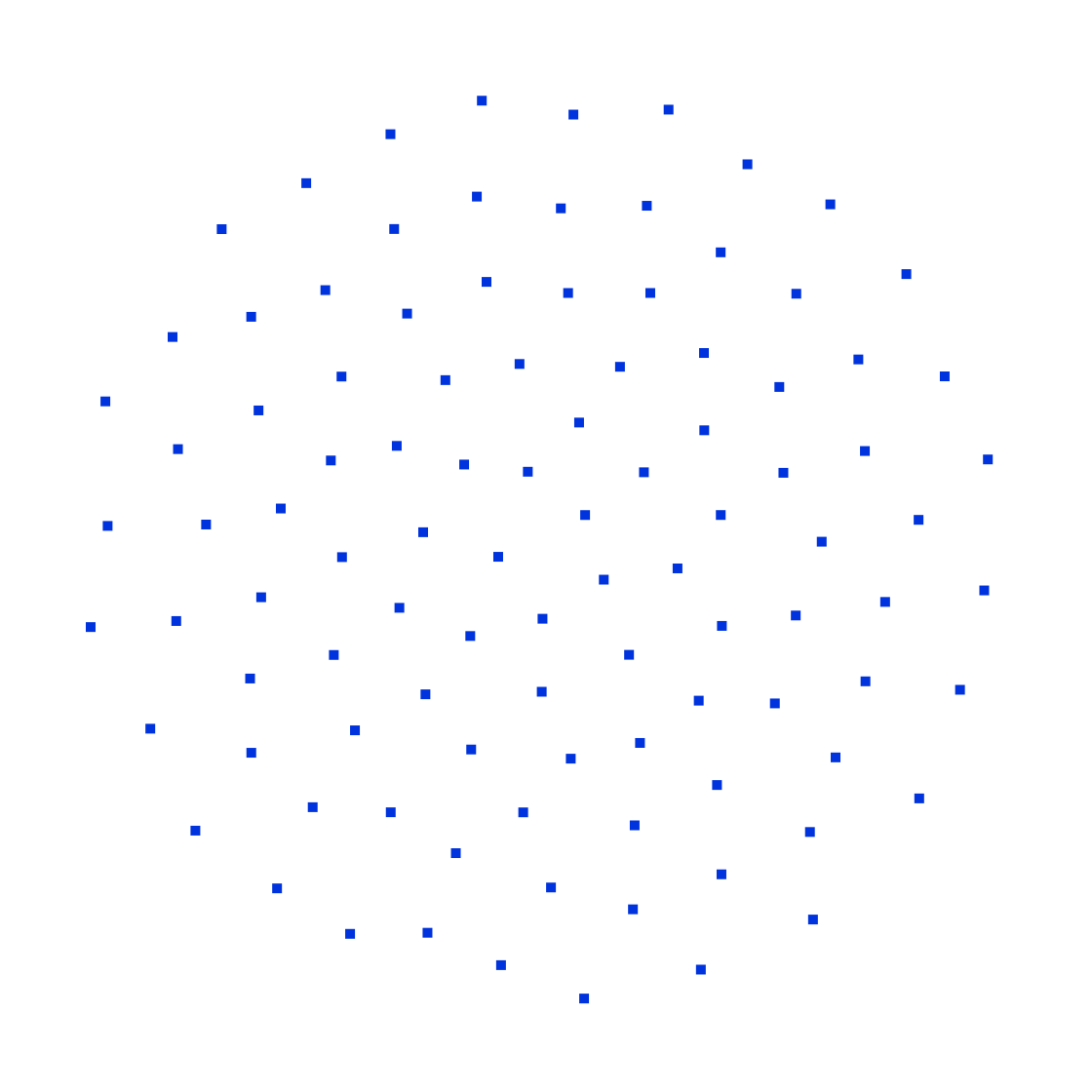## cliqueCircle

``````var cliqueCount = 10;
var cliqueSize = 5;

// create a circle, with `cliqueCount` nodes. Each node is a fully connected
// graph with `cliqueSize` nodes
var graph = require('ngraph.generators').cliqueCircle(cliqueCount, cliqueSize);
``````

## WattsStrogatz

This is a "small world" random graph, generated by Watts and Strogatz model. In this model generator takes three arguments:

• `n` - number of nodes
• `k` - number of edges for each node. Originally node is connected with `k` nearest neighbours on a circle graph
• `b` - probability of an edge rewrite. In other words node changes it's nearest neighbor to a random node inside graph with probability `b`.
``````// Creates graph with 100 nodes, each node is connected with 20 neighbours,
// and probability of neighbour to be outside of local node community is 1%.
var g1 = require('ngraph.generators').wattsStrogatz(100, 20, 0.00);
var g2 = require('ngraph.generators').wattsStrogatz(100, 20, 0.01);
var g3 = require('ngraph.generators').wattsStrogatz(100, 20, 0.10);
var g4 = require('ngraph.generators').wattsStrogatz(100, 20, 0.50);
var g5 = require('ngraph.generators').wattsStrogatz(20, 4, 0.02);
``````

### Watts Strogatz n = 100 k = 20 b = 0.00 (g1):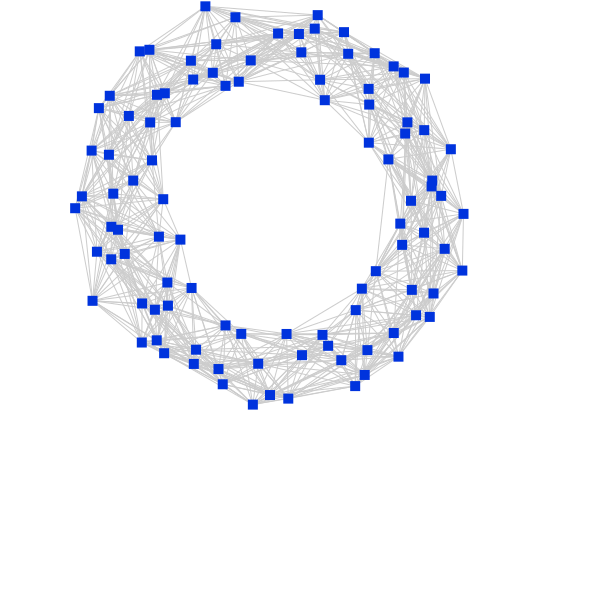### Watts Strogatz n = 100 k = 20 b = 0.01 (g2):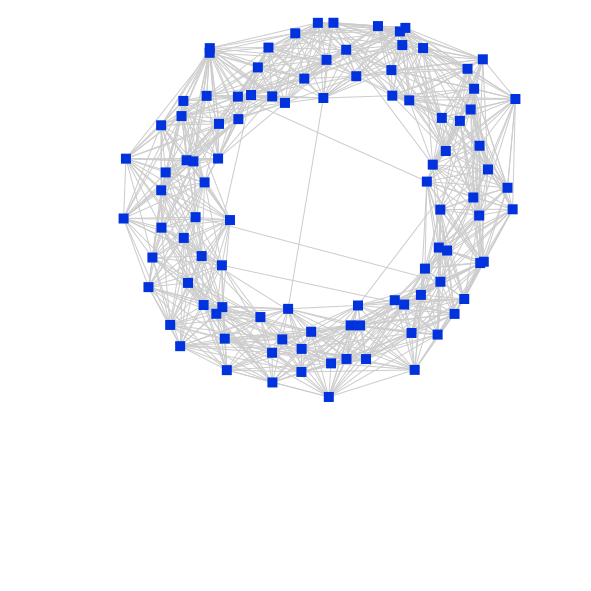### Watts Strogatz n = 100 k = 20 b = 0.10 (g3):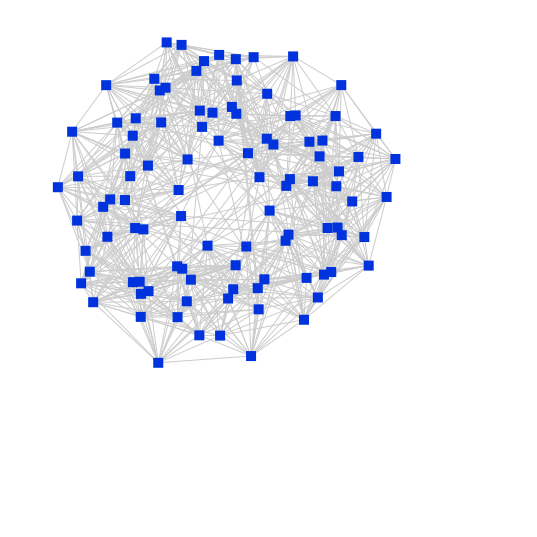### Watts Strogatz n = 100 k = 20 b = 0.50 (g4):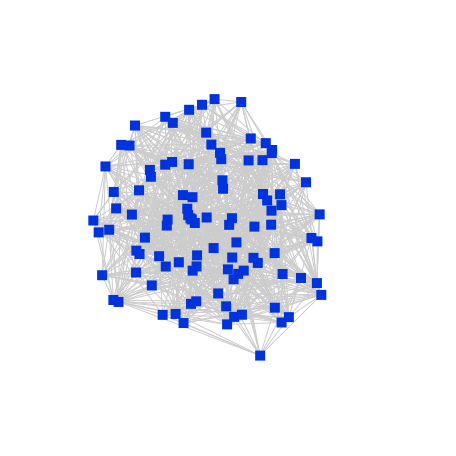### Watts Strogatz n = 20 k = 04 b = 0.02 (g5):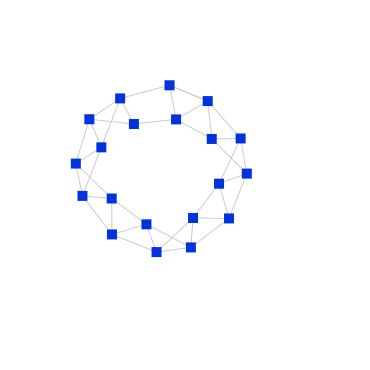# Custom `createGraph()`

By default this library uses `ngraph.graph` module to create new instances of a graph. If you want to use your own module, you can use `factory` method:

``````var generate = require('ngraph.generators').functory(function createGraph() {
// the following methods are required from the createGraph api:
return {
// ...
},
},
getNodesCount() {
}
}
});

// now generators have the same methods as regular ngraph.generators:
var graph = generate.ladder(3);
generate.grid(10, 10);
generate.balancedBinTree(4);
// etc.
``````